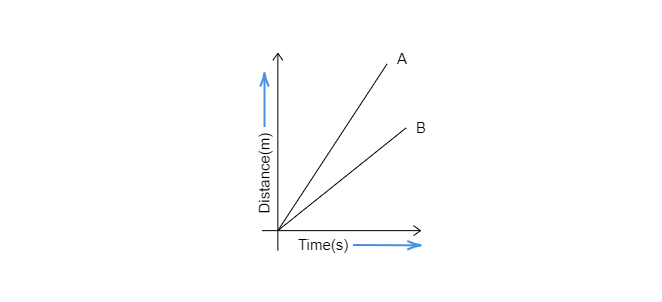# Fig. 13.15 shows the distance-time graph for the motion of two vehicles A and B. Which one of them is moving faster?Fig. 13.15 Distance-time graph for the motion of two cars"

Given:

The distance-time graph for the motion of two vehicles A and B.

To do:

To determine which car A and B is moving faster.

Solution:

To identify the fastest moving car we will have to compare their speeds from the given graph. The higher the speed, the faster the car will move. Speed is the distance covered by a moving object per unit of time.

$\boxed{speed=\frac{distance}{time}}$

Comparison of speeds by graphs:

The greater the slope of a moving object on the graph paper, the greater will be its speed. Lets us observe the slopes of car A and car B in the given graph-

Slopes A and B are representing the speeds of cars A and B.

In the given graph, the slope of car A is more than the slope of car B.

So, speed of the car A is more than car B.

Therefore, Car A is moving faster than Car B.